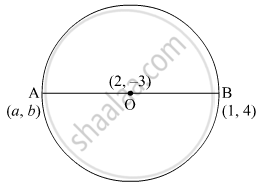# Find the coordinates of a point A, where AB is the diameter of circle whose centre is (2, − 3) and B is (1, 4) - Mathematics

Sum

Find the coordinates of a point A, where AB is the diameter of circle whose centre is (2, − 3) and B is (1, 4)

#### SolutionLet the centre of the circle be O.
Since AB is the diameter so, O is the midpoint of AB.
Thus, using the section formula,

("a" +1)/2 = 2

⇒ a = 4 - 1 = 3

and

("b" + 4)/2 = -3

⇒ b = - 10

So, the coordinate of point A is (3 , -10)

Concept: Section Formula
Is there an error in this question or solution?
Chapter 7: Coordinate Geometry - Exercise 7.2 [Page 167]

#### APPEARS IN

NCERT Class 10 Maths
Chapter 7 Coordinate Geometry
Exercise 7.2 | Q 7 | Page 167

Share